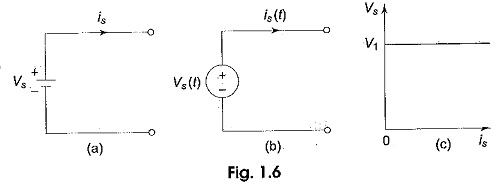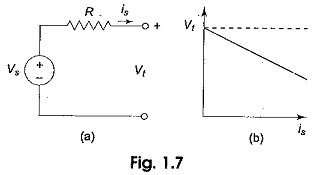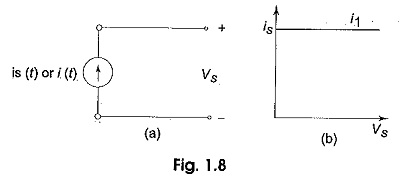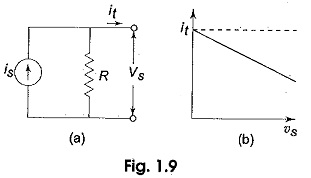# Voltage Current Characteristics

## Voltage Current Characteristics:

According to their terminal Voltage Current Characteristics, electrical Sources of Energy are categorised into ideal voltage sources and ideal current sources. Further they can be divided into independent and dependent sources.

An ideal voltage source is a two-terminal element in which the voltage Vs is completely independent of the current is through its terminals. The representation of ideal constant voltage source is shown in Fig. 1.6(a).If we observe the v — i characteristics for an ideal voltage source as shown in Fig. 1.6(c) at any time, the value of the terminal voltage Vs is constant with respect to the value of current is. Whenever Vs = 0, the voltage source is the same as that of a short circuit. Voltage sources need not have constant magnitude; in many cases the specified voltage may be time-dependent like a sinusoidal waveform. This may be represented as shown in Fig. 1.6(b). In many practical voltage sources, the internal resistance is represented in series with the source as shown in Fig. 1.7(a). In this, the voltage across the terminals falls as the current through it increases, as shown in Fig. 1.7 (b).The terminal voltage Vt depends on the source current as shown in Fig. 1.7(b), where Vt = Vs — is R.

An ideal constant current source is a two-terminal element in which the current is completely independent of the voltage Vs across its terminals. Like voltage sources we can have current sources of constant magnitude is or sources whose current varies with time is(t). The representation of an ideal current source is shown in Fig. 1.8 (a).If we observe the v — i characteristics for an ideal current source as shown in Fig. 1.8(b), at any time the value of the current is is constant with respect to the voltage across it. In many practical current sources, the resistance is in parallel with a source as shown in Fig. 1.9(a). In this the magnitude of the current falls as the voltage across its terminals increases. Its terminal V — i characteristics is shown in Fig. 1.9(b). The terminal current is given by it = is — (Vs/R), where R is the internal resistance of the ideal current source.The two types of ideal sources we have discussed are independent sources for which voltage and current are independent and are not affected by other parts of the circuit.

In the case of dependent sources, the source voltage or current is not fixed, but is dependent on the voltage or current existing at some other location in the circuit.

Dependent or controlled sources are of the following types

(i) voltage controlled voltage source (VCVS)

(ii) current controlled voltage source (CCVS)

(iii) voltage controlled current source (VCCS)

(iv) current controlled current source (CCCS)

These are represented in a circuit diagram by the symbol shown in Fig. 1.10. These types of sources mainly occur in the analysis of equivalent circuits of transistors.

Updated: October 12, 2017 — 10:25 pm

`Please enable JavaScript in your browser to complete this form.Name *FirstLastEmail *Paragraph TextComment`
` `